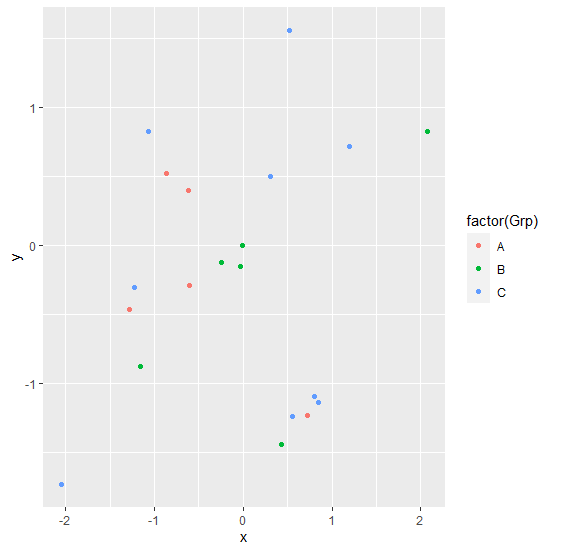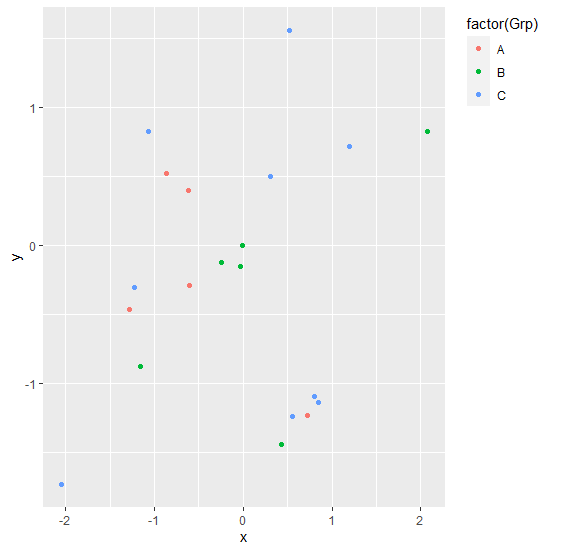# How to display legend on top using ggplot2 in R?

To display legend on top using ggplot2 in R, we can use theme function with legend.justification argument to top.

For Example, if we have a data frame called df that contains three columns say X and Y and F where X and Y are numerical and F is categorical then we can create scatterplot between X and Y with point colored by values in F by using the command given below −

ggplot(df,aes(X,Y))+geom_point(aes(colour=factor(F)))+theme(legend.justification="top")

## Example

Following snippet creates a sample data frame −

x<-rnorm(20)
y<-rnorm(20)
Grp<-sample(LETTERS[1:3],20,replace=TRUE)
df<-data.frame(x,y,Grp)
df

The following dataframe is created

           x             y  Grp
1 -1.066129589  0.828709138 C
2 -1.222825364 -0.306424935 C
3  0.429354500 -1.446665340 B
4 -0.609955977 -0.288351976 A
5  1.200129675  0.716336213 C
6  0.800850596 -1.095220235 C
7  0.517368694  1.561305370 C
8 -0.006456066 -0.002988512 B
9  0.555280469 -1.241763197 C
10 -2.043538292 -1.734706075 C
11 -1.151771052 -0.878394321 B
12  0.845068460 -1.139618835 C
13  2.071684310  0.825474682 B
14 -0.250229200 -0.120597321 B
15 -1.280790787 -0.461785183 A
16 -0.028062183 -0.154095381 B
17 -0.620917924  0.398107075 A
18  0.302128850  0.497374731 C
19  0.719935519 -1.230463223 A
20 -0.862276767  0.518153886 A

To load ggplot2 package and create scatterplot between x and y colored based on Grp values on the above created data frame, add the following code to the above snippet −

x<-rnorm(20)
y<-rnorm(20)
Grp<-sample(LETTERS[1:3],20,replace=TRUE)
df<-data.frame(x,y,Grp)
library(ggplot2)
ggplot(df,aes(x,y))+geom_point(aes(colour=factor(Grp)))

## Output

If you execute all the above given snippets as a single program, it generates the following Output −To create scatterplot between x and y colored based on Grp values with legend on top on the above created data frame, add the following code to the above snippet −

x<-rnorm(20)
y<-rnorm(20)
Grp<-sample(LETTERS[1:3],20,replace=TRUE)
df<-data.frame(x,y,Grp)
library(ggplot2)
ggplot(df,aes(x,y))+geom_point(aes(colour=factor(Grp)))+theme(legend.justification="top")

## Output

If you execute all the above given snippets as a single program, it generates the following Output −